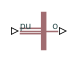# Simplified Synchronous Machine Measurement

Per-unit measurement from simplified synchronous machine

• Library:
• Simscape / Electrical / Electromechanical / Synchronous

•## Description

The Simplified Synchronous Machine Measurement block outputs a per-unit measurement associated with a connected Simplified Synchronous Machine block. The input of the Simplified Synchronous Machine Measurement block connects to the `pu` output port of the Simplified Synchronous Machine block.

You set the Output parameter to a per-unit measurement associated with the simplified synchronous machine. Based on the value you select, the Simplified Synchronous Machine Measurement block:

• Directly outputs the value of an element in the input signal vector

• Calculates the per-unit measurement by using values of elements in the input signal vector in mathematical expressions.

The Simplified Synchronous Machine Measurement block outputs a per-unit measurement from the simplified synchronous machine according to the output value expressions in the table. For example, when you set Output to `Stator d-axis voltage`, the block directly outputs the value of the `pu_ed` element in the input signal vector. However, when you set Output to ```Reactive power```, the block calculates the value from the `pu_ed`, `pu_eq`, `pu_id`, and `pu_iq` elements.

Output Parameter SettingOutput Value Expression

`EMF phase A`

`pu_EMFa`

`EMF phase B`

`pu_EMFb`

`EMF phase C`

`pu_EMFc`

`Electrical torque`

`pu_torque`

`Rotor velocity`

`pu_velocity`

`Stator d-axis voltage`

`pu_vd`

`Stator q-axis voltage`

`pu_vq`

`Stator zero-sequence voltage`

`pu_v0`

`Stator d-axis current`

`pu_id`

`Stator q-axis current`

`pu_iq`

`Stator zero-sequence current`

`pu_i0`

`Apparent power`

$\sqrt{pu_P{t}^{2}+pu_Q{t}^{2}}$

`Real power`

```pu_Pt = (pu_vd*pu_id) + (pu_vq*pu_iq) + 2(pu_v0*pu_i0)```

`Reactive power`

`pu_Qt = (pu_vq*pu_id) – (pu_vd*pu_iq)`

`Terminal voltage`

$\sqrt{\left(pu_v{d}^{2}+\text{​}pu_v{q}^{2}\right)}$

`Terminal current`

$\sqrt{\left(pu_i{d}^{2}+pu_i{q}^{2}\right)}$

`Power factor angle`

`power_factor_angle = atan2(pu_Qt, pu_Pt)`

`Power factor`

`cos(power_factor_angle)`

`Load angle`

`load_angle(rad) = atan2(pu_vd, pu_vq)`

`Rotor electrical angle`

${\theta }_{e}\left(t\right)=N{\theta }_{r}\left(t\right)$

## Ports

### Input

expand all

Physical signal associated with the per-unit measurements from a connected Simplified Synchronous Machine, specified as:

• `pu_EMFa`

• `pu_EMFb`

• `pu_EMFc`

• `pu_torque`

• `pu_velocity`

• `pu_vd`

• `pu_vq`

• `pu_v0`

• `pu_id`

• `pu_iq`

• `pu_i0`

### Output

expand all

Physical signal output port associated with the per-unit measurement, returned as a physical signal.

## Parameters

expand all

Per-unit measurement from simplified synchronous machine. The default value is `EMF phase A`.

## Version History

Introduced in R2020b